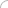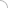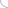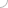Logical Reasoning## Online logical reasoning test - Part 6

##### 51. In this question, a series of numbers is provided. Choose the number which should fill the ‘?’ to follow the pattern of the series.

4, 7, 10, 11, 22, 17, 46, 25, ‘?’

a.) 58
b.) 69
c.) 86
d.) 94
e.) 81

##### 52. In this question, a series of numbers is provided. Choose the number which should fill the ‘?’ to follow the pattern of the series.

7, 11, 16, 23, 25, 35, ‘?’

a.) 34
b.) 36
c.) 38
d.) 42
e.) 45

##### 53. In this question, a series of numbers is provided. Choose the number which should fill the ‘?’ to follow the pattern of the series.

3, 5, 7, 9, 11, 13, 15, 17, ‘?’

a.) 14
b.) 19
c.) 15
d.) 21
e.) 23

##### 54. In this question, a series of numbers is provided. Choose the number which should fill the ‘?’ to follow the pattern of the series.

54. 2, 5, 7, 12, 15, 17, 22, ‘?’

a.) 39
b.) 26
c.) 27
d.) 28
e.) 24

##### 55. In this question, a series of numbers is provided. Choose the number which should fill the ‘?’ to follow the pattern of the series.

5, 14, 27, 44, 65, ‘?’

a.) 88
b.) 90
c.) 109
d.) 130
e.) 121

##### 56. In this question, a series of numbers is provided. Choose the number which should fill the ‘?’ to follow the pattern of the series.

2, 6, 12, 20, 30, ‘?’

a.) 44
b.) 40
c.) 36
d.) 42
e.) 34

##### 57. In this question, a series of numbers is provided. Choose the number which should fill the ‘?’ to follow the pattern of the series.

5, 6, 9, 18, 45, ‘?’

a.) 67.5
b.) 81
c.) 54
d.) 90
e.) 126

##### 58. In this question, a series of numbers is provided. Choose the number which should fill the ‘?’ to follow the pattern of the series.

1, 3, 10, 37, ‘?’

a.) 144
b.) 146
c.) 148
d.) 152
e.) 155

##### 59. In this question, a series of numbers is provided. Choose the number which should fill the ‘?’ to follow the pattern of the series.

4, 7, 12, 19, ‘?’

a.) 25
b.) 26
c.) 27
d.) 29
e.) 28

##### 60. In this question, a series of numbers is provided. Choose the number which should fill the ‘?’ to follow the pattern of the series.

1, 8, 9, 64, 25, 216, ‘?’, ‘?’

a.) 49, 64
b.) 343, 64
c.) 343, 81
d.) 343, 512
e.) 49, 512Aptitude questions